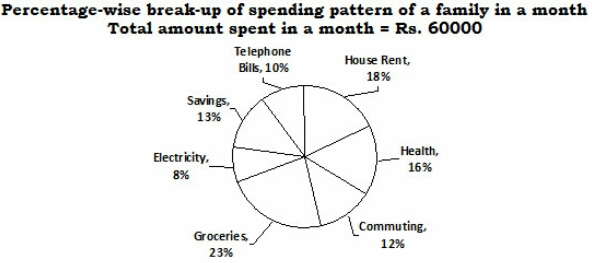# Data Interpretation Quiz - 2 (For SBI PO 2016)

### I. Study The following Pi- Chart and Answer carefully.Click the image to zoom

1. What is the amount spent by the family on Commuting?

1.Rs. 9600
2.Rs. 8400
3.Rs. 7200
4.Rs. 6000
5.None

2. What is the total amount spent by the family on Telephone Bills, Health and Electricity together?

1.Rs. 13800
2.Rs. 18600
3.Rs. 17400
4.Rs. 20400
5.None

3. What is the ratio of the amount spent by the family on Groceries to the amount spent on House Rent?

1.23 : 18
2.13 : 28
3.18 : 23
4.28 : 13
5.None

4. Amount invested by the family on Savings forms what per cent of the amount spent on Health?

1.123
2.81.25
3.120.50
4.85.75
5.None

5. Total amount spent by the family on Commuting and Telephone Bills together forms approximately . what per cent of the amount spent on Groceries?

1.153
2.148
3.135
4.112
5.96

### II. Study Following table and answer carefully .Click the image to zoom

6. Total population of city M for all the given years together is what percent of the total population of city P for all the given years together ? (rounded off to nearest integer)

1.32
2.36
3.38
4.29
5.28

7. What is the respective ratio between total population of cities K, M & N together in 2004 & totalpopulation of cities L. J & P together in 2001 ?

1.23 : 25
2.21 : 23
3.21 : 25
4.21 : 22
5.22 : 23

8. If in 2004 7% of the population of city N was illiterate & 6% of the population of city K was illiterate what is the respective ratio between illiterate populations of cities N & K ?

1.91 : 68
2.89 : 66
3.92 : 67
4.81 : 67
5.91 : 66

9. Population of city M in 2002 is approximately what percent of the total population of all the six cities in that year ?

1.11
2.9
3.14
4.7
5.12

10. In 2003. in city J. 48% of the population was female population & for city L it was 47%. What was the total male population (in lakhs) of these two cities in 2003 ?

1.27.44
2.23.44
3.22.47
4.24.24
5.24.74

#### Must follow

Data Interpretation Guide here

1) (12*60000)/100 =7200

2) (10+16+8)*(60000/100)=20400

3) 23 : 18

4) (13*100)/16 = 81.25

5) ((12+10)/23)*100 = 95.65 = 96%

6) M = 9+12+13+15+17+19 = 85

P = 31+35+36+38+39+44 = 223

Percentage = (85*100)/223 = 38.11 = 38%

7) K,M & N population in 2004 = 22 + 15 + 26 = 63

L,J & P population in 2004 = 23+12+31 = 66

Ratio = 63 : 66 = 21: 22

8) N:K = (26*7) : (22*6) = 91: 66

9) In 2002 All 6 cities population = 16+18+28+12+22+35 = 131

City M population in  = 12

Percentage = (12*100)/131 = 9.16 = 9%

10) Male population in J 2003 = (52*17)/100 = 8.84

Male population in L 2003 = (53*30)/100 = 15.90

Total = 8.84+15.90=24.74 Lakh